# Solving MultiStep Equations Solve the Equations Below 1

• Slides: 9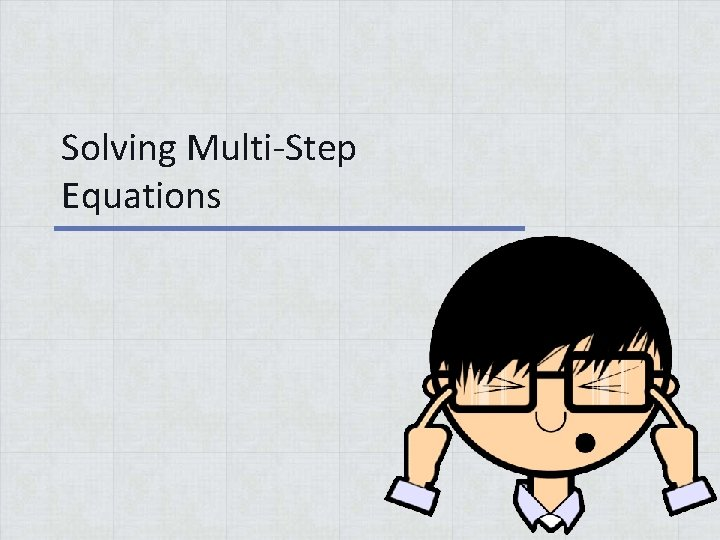Solving Multi-Step EquationsSolve the Equations Below 1. 2 h + 6 = 20 5. 4 r + 14 = 26 2. 3 n + 11 = 26 6. 8 f + 21 = 37 3. 4 w + 5 = 29 7. 6 a + 32 = 44 4. 7 t + 3 = 31 8. 9 p + 32 = 59 h=7 n=5 w=6 t=4 11/24/2020 r=3 f=2 a=2 p=3 www. numberbender. com 2SOLVING LINEAR EQUATIONS 11/24/2020 www. numberbender. com 3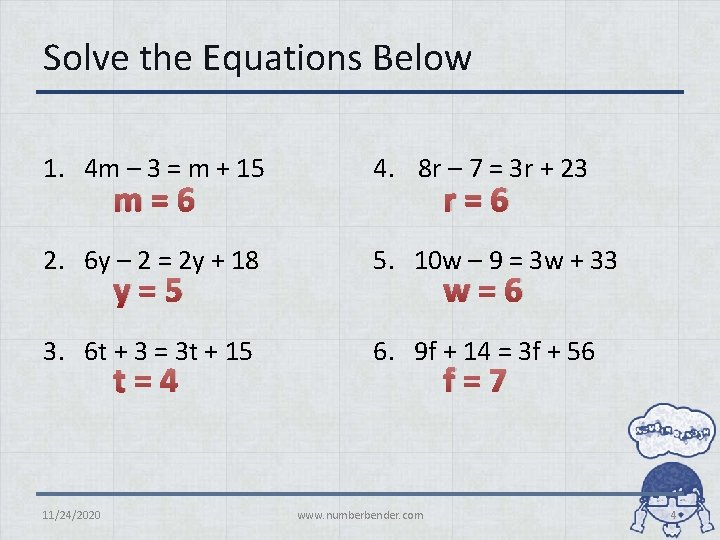Solve the Equations Below 1. 4 m – 3 = m + 15 4. 8 r – 7 = 3 r + 23 2. 6 y – 2 = 2 y + 18 5. 10 w – 9 = 3 w + 33 3. 6 t + 3 = 3 t + 15 6. 9 f + 14 = 3 f + 56 m=6 y=5 t=4 11/24/2020 r=6 w=6 f=7 www. numberbender. com 4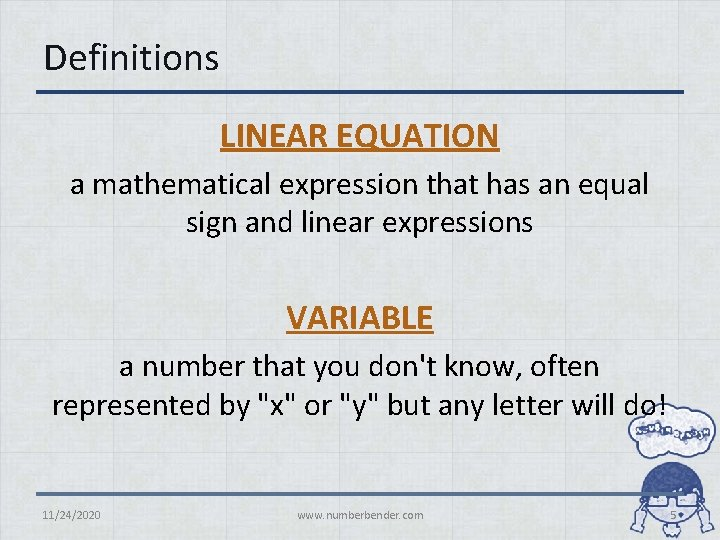Definitions LINEAR EQUATION a mathematical expression that has an equal sign and linear expressions VARIABLE a number that you don't know, often represented by "x" or "y" but any letter will do! 11/24/2020 www. numberbender. com 5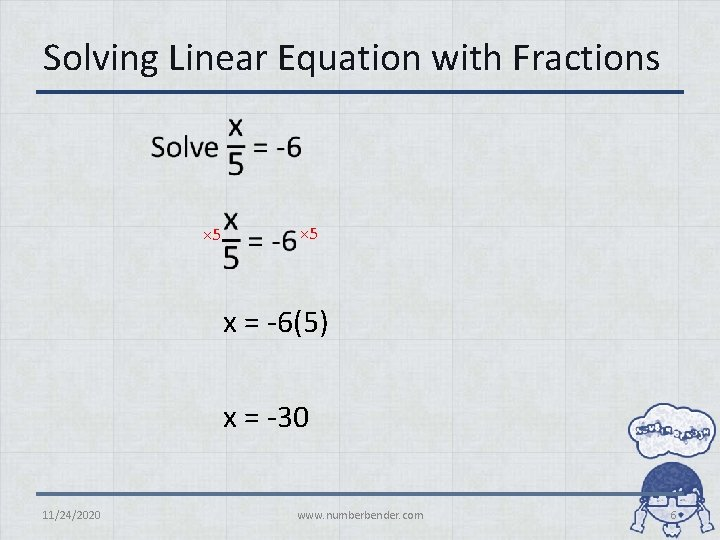Solving Linear Equation with Fractions • × 5 x = -6(5) x = -30 11/24/2020 www. numberbender. com 6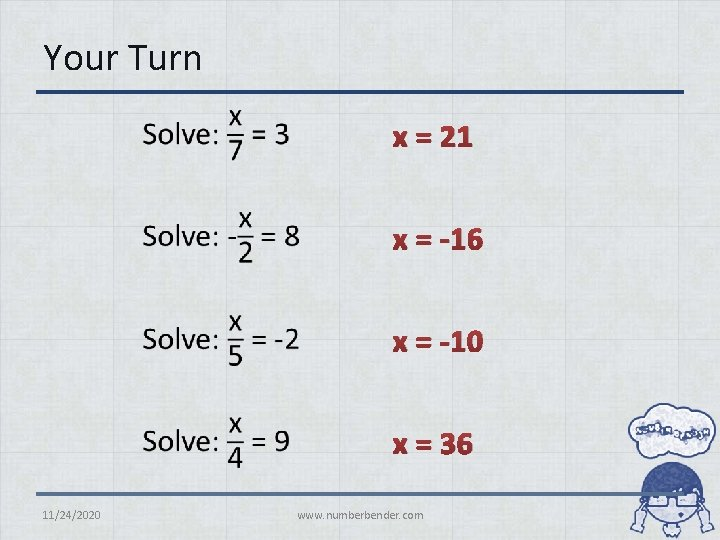Your Turn • 11/24/2020 x = 21 x = -16 x = -10 x = 36 www. numberbender. comLinear Equations with Fractions 11/24/2020 www. numberbender. com 8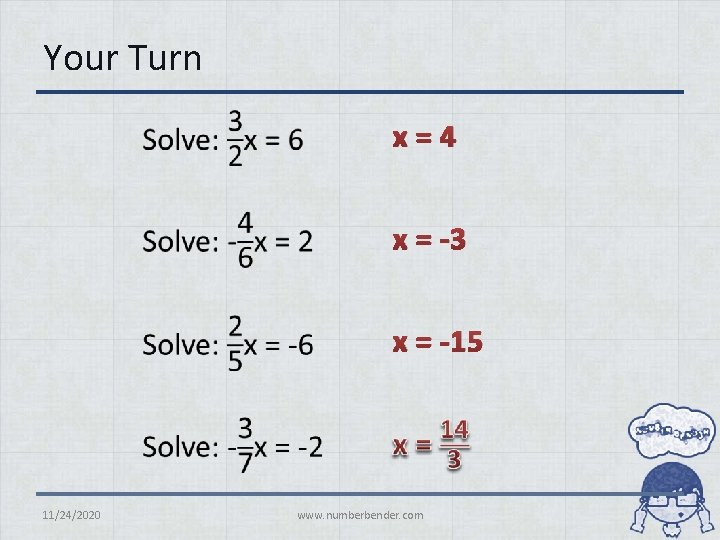Your Turn • 11/24/2020 x=4 x = -3 x = -15 www. numberbender. com